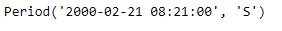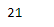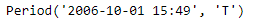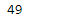# Python | Pandas Period.minute

• Last Updated : 06 Jan, 2019

Python is a great language for doing data analysis, primarily because of the fantastic ecosystem of data-centric python packages. Pandas is one of those packages and makes importing and analyzing data much easier.

Pandas` Period.minute` attribute return an integer value. The returned value represents the number of minutes in the given Period object.

Attention geek! Strengthen your foundations with the Python Programming Foundation Course and learn the basics.

To begin with, your interview preparations Enhance your Data Structures concepts with the Python DS Course. And to begin with your Machine Learning Journey, join the Machine Learning - Basic Level Course

Syntax : Period.minute

Parameters : None

Return : number of minutes

Example #1: Use `Period.minute `attribute to find the minute value present in the given Period object.

 `# importing pandas as pd``import` `pandas as pd`` ` `# Create the Period object``prd ``=` `pd.Period(freq ``=``'S'``, year ``=` `2000``, month ``=` `2``, day ``=` `21``, hour ``=` `8``, minute ``=` `21``)`` ` `# Print the Period object``print``(prd)`

Output :Now we will use the `Period.minute` attribute to find out the minute value present in prd object.

 `# find minute value``prd.minute`

Output :As we can see in the output, the `Period.minute `attribute has returned 21 indicating the minute value present in the given period object is 21.

Example #2: Use `Period.minute` attribute to find the minute value present in the given Period object.

 `# importing pandas as pd``import` `pandas as pd`` ` `# Create the Period object``prd ``=` `pd.Period(freq ``=``'T'``, year ``=` `2006``, month ``=` `10``,``                            ``hour ``=` `15``, minute ``=` `49``)`` ` `# Print the Period object``print``(prd)`

Output :Now we will use the `Period.minute` attribute to find out the minute value present in prd object.

 `# find minute value``prd.minute`

Output :As we can see in the output, the `Period.minute `attribute has returned 49 indicating the minute value present in the given period object is 49.

My Personal Notes arrow_drop_up